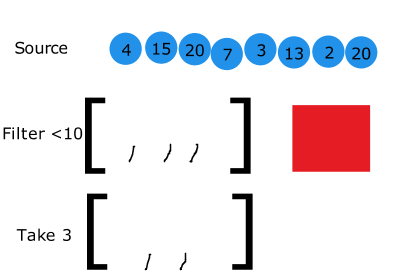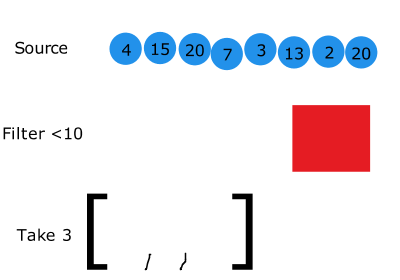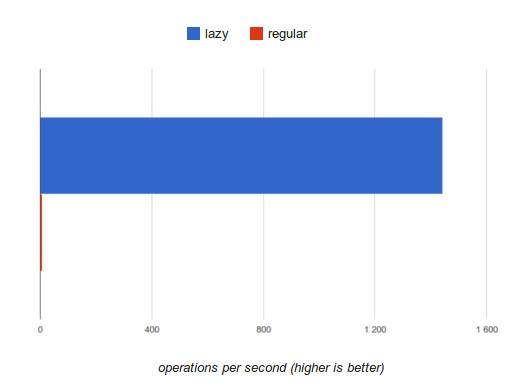## 惰性计算

``````var len = getLength();
for(var i = 0; i < len; i++) {
operation(); // <- 10毫秒 - 如何优化到9毫秒?!
}
``````

``````function priceLt(x) {
return function(item) { return item.price < x; };
}
var gems = [
{ name: 'Sunstone', price: 4  },
{ name: 'Amethyst', price: 15 },
{ name: 'Prehnite', price: 20 },
{ name: 'Sugilite', price: 7  },
{ name: 'Diopside', price: 3  },
{ name: 'Feldspar', price: 13 },
{ name: 'Dioptase', price: 2  },
{ name: 'Sapphire', price: 20 }
];

var chosen = _(gems).filter(priceLt(10)).take(3).value();
````````````// 99,999 张照片
var phoneNumbers = [5554445555, 1424445656, 5554443333, … ×99,999];

// 返回包含 "55" 的照片
function contains55(str) {
return str.contains("55");
};

// 取 100 张包含 "55" 的照片
var r = _(phoneNumbers).map(String).filter(contains55).take(100);
``````## Pipelining

``````var result = _(source).map(func1).map(func2).map(func3).value();
``````

``````var result = [], temp1 = [], temp2 = [], temp3 = [];

for(var i = 0; i < source.length; i++) {
temp1[i] = func1(source[i]);
}

for(i = 0; i < source.length; i++) {
temp2[i] = func2(temp1[i]);
}

for(i = 0; i < source.length; i++) {
temp3[i] = func3(temp2[i]);
}
result = temp3;
``````

``````var result = [];
for(var i = 0; i < source.length; i++) {
result[i] = func3(func2(func1(source[i])));
}
``````

## 延迟执行

``````var wallet = _(assets).filter(ownedBy('me'))
.pluck('value')
.reduce(sum);

\$json.get("/new/assets").success(function(data) {
assets.push.apply(assets, data); // 更新我的资金
wallet.value(); // 返回我钱包的最新的总额
});
``````

## Wrap up